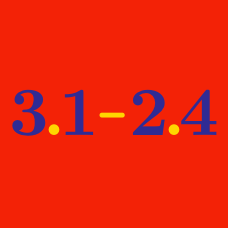Everyday Math

# Scientific Notation

Which of the following correctly expresses $3.102$ in scientific notation?

Which of the following numbers is equal to $2 . 174 \times 10 ^ 6$?

Which of the following correctly expresses $0. 3 134$ in scientific notation?

Which of the following numbers is equal to $2 . 204 \times 10 ^ {-2}$?

Which of the following can not be a valid representation of $464,700$ in scientific notation?

×